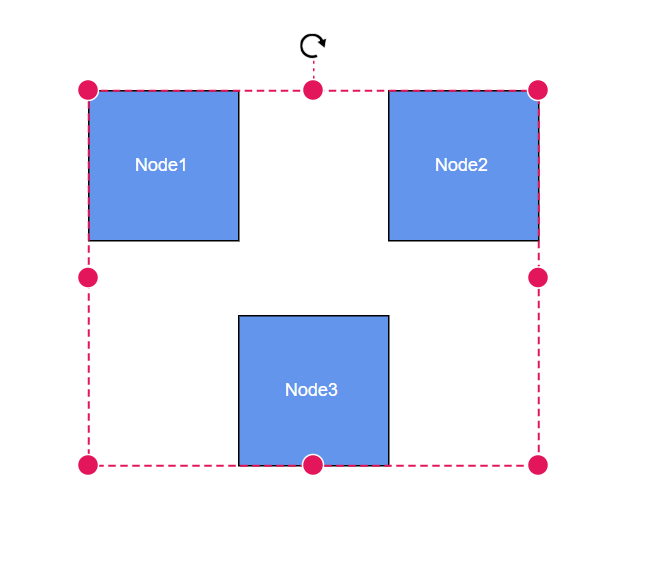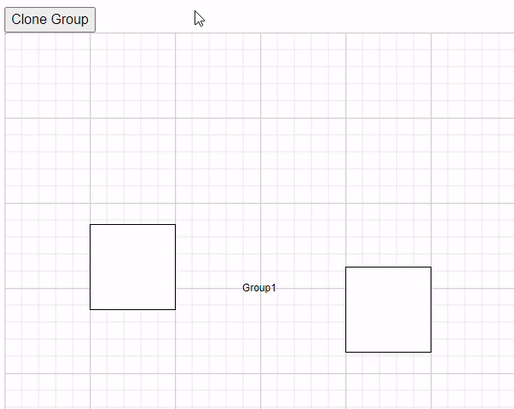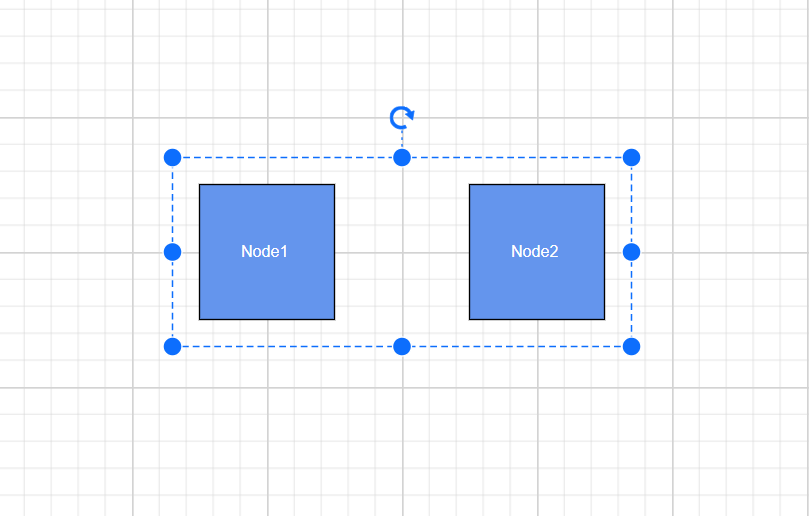# NodeGroup in Blazor Diagram Component

22 Jun 202324 minutes to read

NodeGroup is used to cluster multiple nodes and connectors into a single element. It acts like a container for its children (nodes, nodegroups, and connectors). Every change made to the node group also affects the children. Child elements can be edited individually.

## How to add nodeGroup while initialize the diagram

A node group can be added to the diagram model through Nodes collection. To define an object as node group, add the child objects to the Children collection of the node group. The following code illustrates how to create a node group.

• While creating node group, its child node needs to be declared before the node group declaration.

• Add a node to the existing node group child by using the Group method.

• The nodegroup’s Ungroup method is used to define whether the node group can be ungrouped or not.

• A node group can be added into a child of another node group.

To create a group using Nodes and Connectors in the Blazor Diagram, refer to the below video link,

``````@using Syncfusion.Blazor.Diagram

@* Initialize the diagram with NodeCollection *@
<SfDiagramComponent Height="500px" @ref="diagram" Nodes="@nodes">
<SnapSettings>
<HorizontalGridLines LineColor="white" LineDashArray="2,2"></HorizontalGridLines>
<VerticalGridLines LineColor="white" LineDashArray="2,2"></VerticalGridLines>
</SnapSettings>
</SfDiagramComponent>

@code
{
SfDiagramComponent diagram;
DiagramObjectCollection<Node> nodes = new DiagramObjectCollection<Node>();

protected override void OnInitialized()
{
Node node1 = createNode("node1", 100, 100, "Node1");
Node node2 = createNode("node2", 300, 100, "Node2");
Node node3 = createNode("node3", 200, 250, "Node3");
NodeGroup groupNode = new NodeGroup();
// Grouping node 1 and node 2 into a single nodegroup.
groupNode.Children = new string[] { "node1", "node2" };
}

public Node createNode(string id, double offsetX, double offsetY, string content)
{
Node node = new Node()
{
ID = id,
OffsetX = offsetX,
OffsetY = offsetY,
Height = 100,
Width = 100,
Style = new ShapeStyle() { Fill = "#6495ED" }
};
ShapeAnnotation annotation = new ShapeAnnotation
{
ID = "annotation1",
Content = content,
Style = new TextStyle()
{
Color = "white",
Fill = "transparent",
StrokeColor = "None"
},
};
node.Annotations = new DiagramObjectCollection<ShapeAnnotation>()
{
annotation
};
return node;
}

protected override async Task OnAfterRenderAsync(bool firstRender)
{
if (firstRender)
{
diagram.SelectAll();
// Adding the third node into the existing nodegroup.
diagram.Group();
}
}
}``````The following code illustrates how to ungroup at runtime.

``````@using Syncfusion.Blazor.Diagram

@* Initialize the diagram with nodes *@
<SfDiagramComponent Height="500px" @ref="diagram" Nodes="@nodes" />

@code
{
SfDiagramComponent diagram;
DiagramObjectCollection<Node> nodes = new DiagramObjectCollection<Node>();
protected override void OnInitialized()
{
Node node1 = createNode("node1", 100, 100, "Node1");
Node node2 = createNode("node2", 300, 100, "Node2");
NodeGroup groupNode = new NodeGroup();
// Grouping node 1 and node 2 into a single nodegroup.
groupNode.Children = new string[] { "node1", "node2" };
}

public Node createNode(string id, double offsetX, double offsetY, string content)
{
Node node = new Node()
{
ID = id,
OffsetX = offsetX,
OffsetY = offsetY,
Height = 100,
Width = 100,
Style = new ShapeStyle() { Fill = "#6495ED" }
};
ShapeAnnotation annotation = new ShapeAnnotation
{
ID = "annotation1",
Content = content,
Style = new TextStyle()
{
Color = "white",
Fill = "transparent",
StrokeColor = "None"
},
};
node.Annotations = new DiagramObjectCollection<ShapeAnnotation>()
{
annotation
};
return node;
}

protected override async Task OnAfterRenderAsync(bool firstRender)
{
if (firstRender)
{
diagram.SelectAll();
// Ungroup the selected group into nodes.
diagram.Ungroup();
}
}
}``````

### How to clone the group of node at runtime

Clone is a virtual method of the node that is used to create a copy of a diagram object. After cloning, we need to set the ID for cloned nodes. The following code demonstrates how to clone the group of nodes during runtime.

``````@using Syncfusion.Blazor.Diagram
@using System.Collections.ObjectModel
@inject IJSRuntime js
@using Syncfusion.Blazor.Buttons

<SfButton Content="CloneGroup" OnClick="@CloneGroup" />
<SfDiagramComponent @ref="diagram" Width="50%" Height="500px" @bind-Nodes="NodeCollection"></SfDiagramComponent>

@functions
{

SfDiagramComponent diagram;
public DiagramObjectCollection<Node> NodeCollection = new DiagramObjectCollection<Node>();
protected override void OnInitialized()
{
Node node1 = new Node()
{
OffsetX = 100,
OffsetY = 150,
Height = 100,
Width = 100,
ID = "node1",

};
Node node2 = new Node()
{
OffsetX = 400,
OffsetY = 200,
Height = 100,
Width = 100,
ID = "node2",

};
NodeGroup group1 = new NodeGroup()
{
ID = "group1",
Children = new string[] { "node1", "node2" },
OffsetX = 300,
OffsetY = 300,
Annotations = new DiagramObjectCollection<ShapeAnnotation>()
{
new ShapeAnnotation()
{
Content = "Group1"
}
},
};
}
{
Node node2 = NodeCollection.Clone() as Node;
node2.ID = RandomId();
Node node3 = NodeCollection.Clone() as Node;
node3.ID = RandomId();
Node node4 = NodeCollection.Clone() as Node;
node4.ID = RandomId();
string[] array = { node2.ID, node3.ID };
(node4 as NodeGroup).Children = array;
node4.OffsetX += 25;
node4.OffsetY += 25;
await diagram.AddDiagramElements(new DiagramObjectCollection<NodeBase>() { node2, node3, node4 });
}

internal string RandomId()
{
Random random = new Random();
const string chars = "ABCDEFGHIJKLMNOPQRSTUVWXTZabcdefghiklmnopqrstuvwxyz";
#pragma warning disable CA5394 // Do not use insecure randomness
return new string(Enumerable.Repeat(chars, 5)
.Select(s => s[random.Next(s.Length)]).ToArray());
#pragma warning restore CA5394 // Do not use insecure randomness
}

}``````The Padding property of a group node defines the spacing between the group node’s edges and its children.

The following code illustrates how to add Padding to the node group.

``````@using Syncfusion.Blazor.Diagram

@* Initialize the diagram with NodeCollection *@
<SfDiagramComponent Height="500px" Nodes="@nodes" />

@code
{
DiagramObjectCollection<Node> nodes = new DiagramObjectCollection<Node>();
NodeGroup groupNode = new NodeGroup();

protected override void OnInitialized()
{
Node node1 = createNode("node1", 100, 100, "Node1");
Node node2 = createNode("node2", 300, 100, "Node2");
// Grouping node one and node two into a single node-group.
groupNode.Children = new string[] { "node1", "node2" };
groupNode.Padding = new DiagramThickness() { Left=20, Right= 20, Top=20, Bottom=20 };
}

public Node createNode(string id, double offsetX, double offsetY, string content)
{
Node node = new Node()
{
ID = id,
OffsetX = offsetX,
OffsetY = offsetY,
Height = 100,
Width = 100,
Style = new ShapeStyle() { Fill = "#6495ED" }
};
ShapeAnnotation annotation = new ShapeAnnotation()
{
ID = "annotation1",
Content = content,
Style = new TextStyle()
{
Color = "white",
Fill = "transparent",
StrokeColor = "None"
},
};
node.Annotations = new DiagramObjectCollection<ShapeAnnotation>()
{
annotation
};
return node;
}
}``````## How to add nodeGroup at runtime

A node group can be added at runtime by using Nodes collection of diagram.

The following code illustrates how a node group is added at runtime.

``````@using Syncfusion.Blazor.Diagram
@using Syncfusion.Blazor.Buttons

@* Initialize the diagram with NodeCollection *@
<SfDiagramComponent Height="500px" Nodes="@nodes" />

@code
{
DiagramObjectCollection<Node> nodes = new DiagramObjectCollection<Node>();
NodeGroup groupNode = new NodeGroup();

protected override void OnInitialized()
{
Node node1 = createNode("node1", 100, 100, "Node1");
Node node2 = createNode("node2", 300, 100, "Node2");
// Grouping node 1 and node 2 into a single node-group.
groupNode.Children = new string[] { "node1", "node2" };
}

public Node createNode(string id, double offsetX, double offsetY, string content)
{
Node node = new Node()
{
ID = id,
OffsetX = offsetX,
OffsetY = offsetY,
Height = 100,
Width = 100,
Style = new ShapeStyle() { Fill = "#6495ED" }
};
ShapeAnnotation annotation = new ShapeAnnotation()
{
ID = "annotation1",
Content = content,
Style = new TextStyle()
{
Color = "white",
Fill = "transparent",
StrokeColor = "None"
},
};
node.Annotations = new DiagramObjectCollection<ShapeAnnotation>()
{
annotation
};
return node;
}

{
}
}``````

• Also, you can add the child to the node group through AddChild method. The following code illustrates how to add child to the existing node group through AddChild method.
``````@using Syncfusion.Blazor.Diagram
@using Syncfusion.Blazor.Buttons

@* Initialize the diagram with nodes *@
<SfDiagramComponent @ref="@diagram" Height="500px" Nodes="@nodes"/>

@code
{
SfDiagramComponent diagram;
DiagramObjectCollection<Node> nodes = new DiagramObjectCollection<Node>();
NodeGroup groupNode = new NodeGroup();

protected override void OnInitialized()
{
Node node1 = createNode("node1", 100, 100, "Node1");
Node node2 = createNode("node2", 300, 100, "Node2");
// Grouping node 1 and node 2 into a single node-group.
groupNode.Children = new string[] { "node1", "node2" };
}

public Node createNode(string id, double offsetX, double offsetY, string content)
{
Node node = new Node()
{
ID = id,
OffsetX = offsetX,
OffsetY = offsetY,
Height = 100,
Width = 100,
Style = new ShapeStyle() { Fill = "#6495ED" }
};
ShapeAnnotation annotation = new ShapeAnnotation()
{
ID = "annotation1",
Content = content,
Style = new TextStyle()
{
Color = "white",
Fill = "transparent",
StrokeColor = "None"
},
};
node.Annotations = new DiagramObjectCollection<ShapeAnnotation>()
{
annotation
};
return node;
}

{
NodeGroup group = diagram.SelectionSettings.Nodes as NodeGroup;
Node node = new Node()
{
ID = "node" + nodes.Count.ToString(),
OffsetX = 250,
OffsetY = 250,
Width = 50,
Height = 50,
Style = new ShapeStyle() { Fill = "#6495ED" },
Annotations = new DiagramObjectCollection<ShapeAnnotation>()
{
new ShapeAnnotation()
{
Content = "Node" + nodes.Count.ToString(),
Style = new TextStyle()
{
Color = "white",
Fill = "transparent",
StrokeColor = "None"
},
}
}
};
}
}``````

## How to update nodeGroup’s position at runtime

You can change the position of the node group similar to node. For more information about node positioning, refer to Positioning.

``````@using Syncfusion.Blazor.Diagram
@using Syncfusion.Blazor.Buttons

<SfButton Content="UpdatePosition" OnClick="@UpdatePosition" />
@* Initialize the diagram with NodeCollection *@
<SfDiagramComponent Height="500px" Nodes="@nodes" />

@code
{
DiagramObjectCollection<Node> nodes = new DiagramObjectCollection<Node>();
NodeGroup groupNode = new NodeGroup();

protected override void OnInitialized()
{
nodes = new DiagramObjectCollection<Node>();
Node node1 = createNode("node1", 100, 100, "Node1");
Node node2 = createNode("node2", 300, 100, "Node2");
// Grouping node 1 and node 2 into a single NodeGroup.
groupNode.Children = new string[] { "node1", "node2" };
}

public Node createNode(string id, double offsetX, double offsetY, string content)
{
Node node = new Node()
{
ID = id,
OffsetX = offsetX,
OffsetY = offsetY,
Height = 100,
Width = 100,
Style = new ShapeStyle() { Fill = "#6495ED" }
};
ShapeAnnotation annotation = new ShapeAnnotation
{
ID = "annotation1",
Content = content,
Style = new TextStyle()
{
Color = "white",
Fill = "transparent",
StrokeColor = "None"
},
};
node.Annotations = new DiagramObjectCollection<ShapeAnnotation>()
{
annotation
};
return node;
}

private void UpdatePosition()
{
nodes.OffsetX = 500;
nodes.OffsetY = 200;
}
}``````

## How to change appearance of the node group

You can change the appearance of the node group similar to node. For more information about node appearance, refer to Appearance.

## Interact with the node group

You can edit the node group and its children at runtime. You can interact with the nodegroup as like the node interaction like resize, rotate and drag. For more information about node interaction, refer to Interaction.

### Selecting a NodeGroup

When a child element of any node group is clicked, its contained node group is selected instead of the child element. With consecutive clicks on the selected element, selection is changed from top to bottom in the hierarchy of parent nodegroup to its children.

## GroupNode Flip

The Flip is performed to give the mirrored image of the original element.